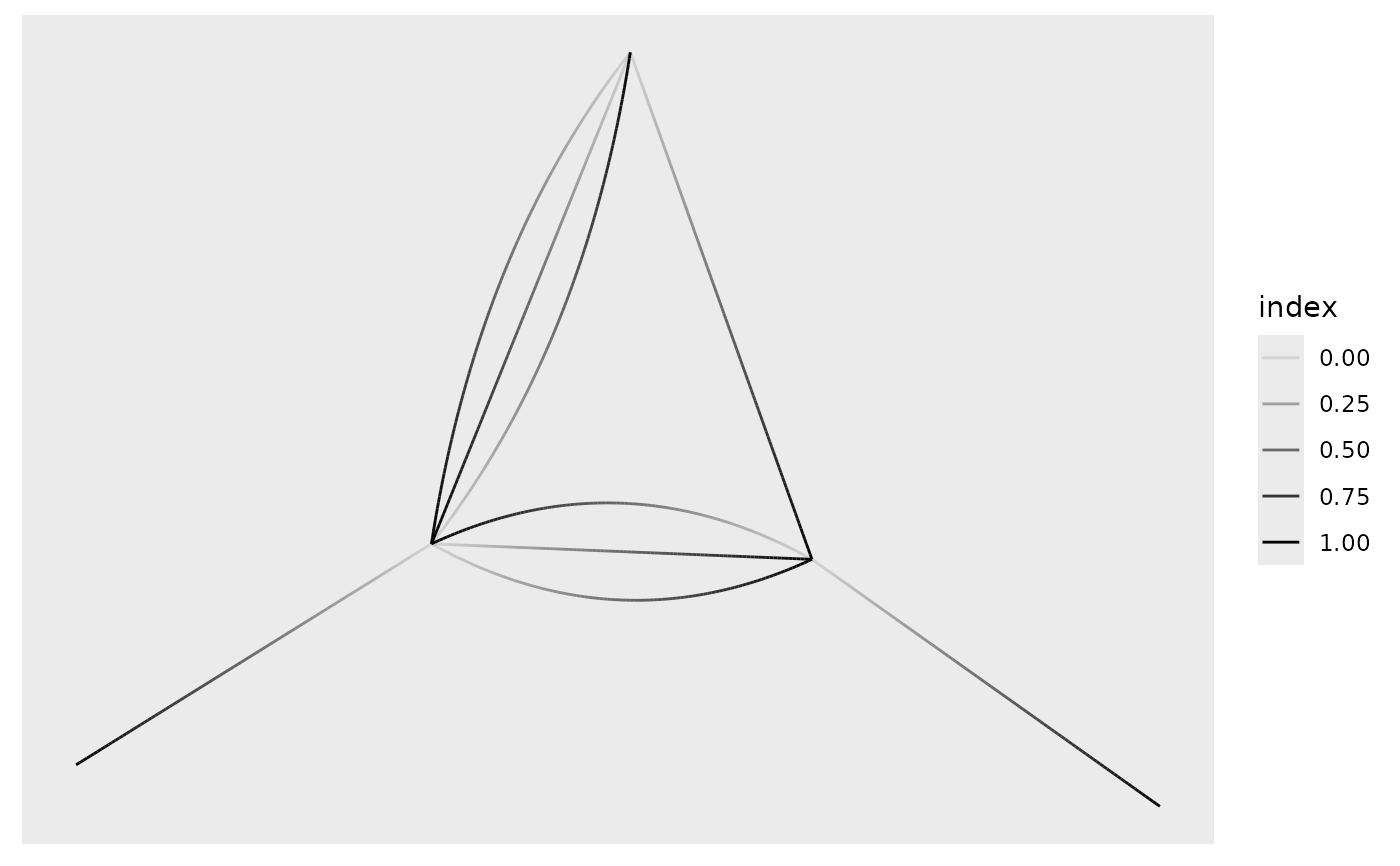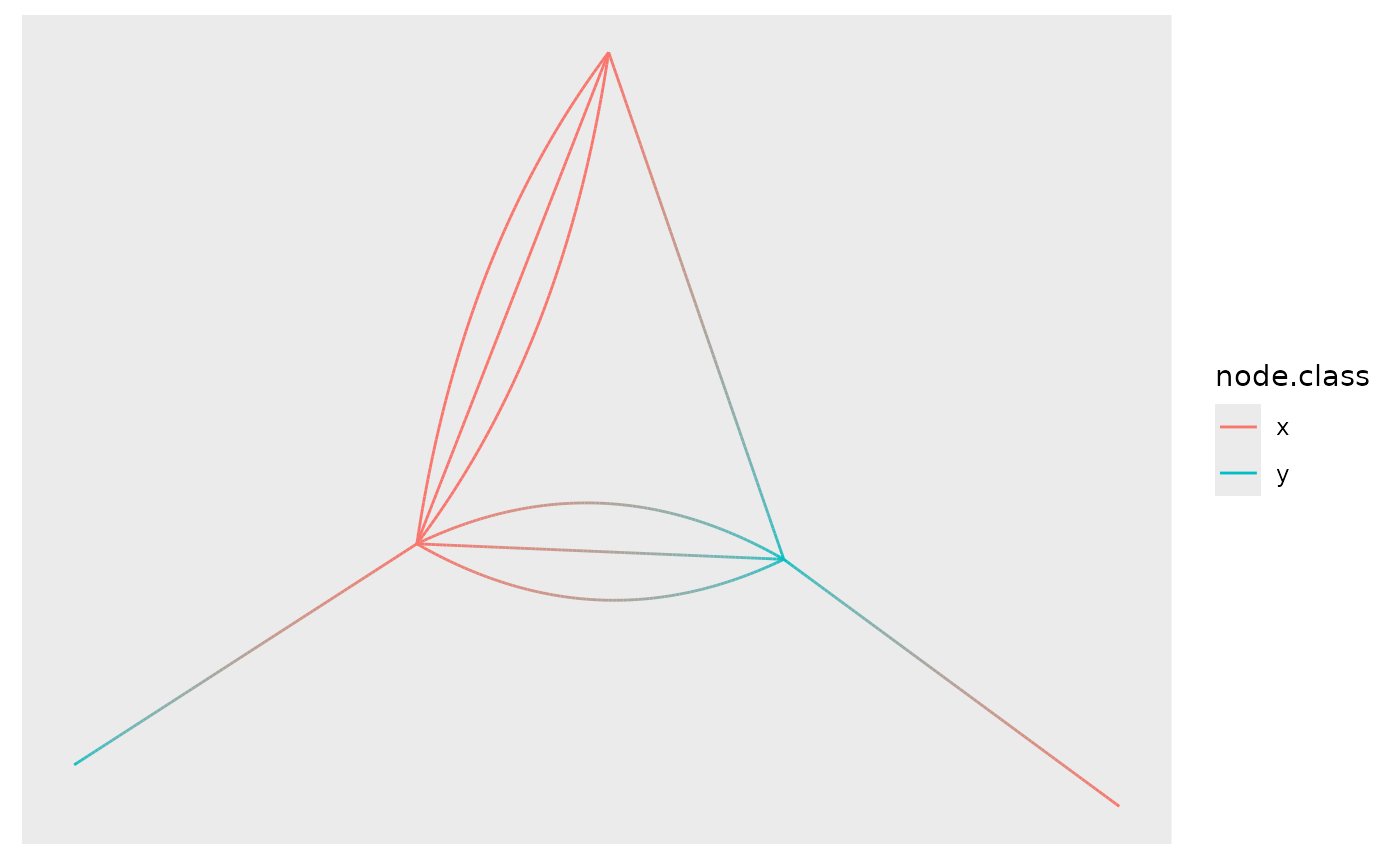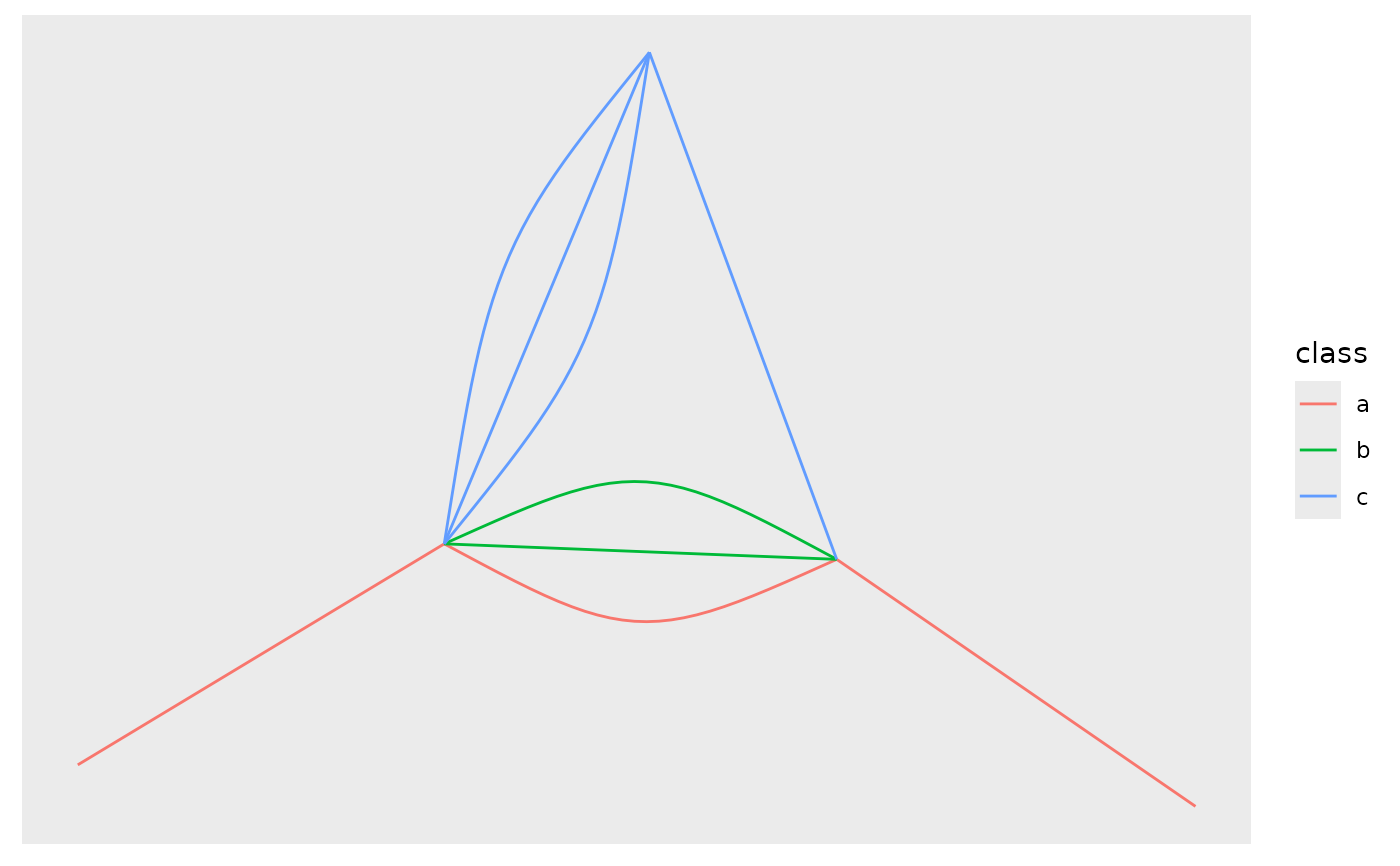This geom draws edges as cubic beziers with the control point positioned half-way between the nodes and at an angle dependent on the presence of parallel edges. This results in parallel edges being drawn in a non-overlapping fashion resembling the standard approach used in igraph::plot.igraph(). Before calculating the curvature the edges are sorted by direction so that edges going the same way will be adjacent. This geom is currently the only choice for non-simple graphs if edges should not be overplotted.

geom_edge_fan(
mapping = NULL,
data = get_edges(),
position = "identity",
arrow = NULL,
strength = 1,
n = 100,
lineend = "butt",
linejoin = "round",
linemitre = 1,
label_colour = "black",
label_alpha = 1,
label_parse = FALSE,
check_overlap = FALSE,
angle_calc = "rot",
force_flip = TRUE,
label_dodge = NULL,
label_push = NULL,
show.legend = NA,
...,
)

geom_edge_fan2(
mapping = NULL,
data = get_edges("long"),
position = "identity",
arrow = NULL,
strength = 1,
n = 100,
lineend = "butt",
linejoin = "round",
linemitre = 1,
label_colour = "black",
label_alpha = 1,
label_parse = FALSE,
check_overlap = FALSE,
angle_calc = "rot",
force_flip = TRUE,
label_dodge = NULL,
label_push = NULL,
show.legend = NA,
...,
)

geom_edge_fan0(
mapping = NULL,
data = get_edges(),
position = "identity",
arrow = NULL,
strength = 1,
lineend = "butt",
show.legend = NA,
...,
)

## Arguments

mapping

Set of aesthetic mappings created by ggplot2::aes() or ggplot2::aes_(). By default x, y, xend, yend, group and circular are mapped to x, y, xend, yend, edge.id and circular in the edge data.

data

The return of a call to get_edges() or a data.frame giving edges in correct format (see details for for guidance on the format). See get_edges() for more details on edge extraction.

position

Position adjustment, either as a string, or the result of a call to a position adjustment function.

arrow

Arrow specification, as created by grid::arrow().

strength

Modify the width of the fans strength > 1 will create wider fans while the reverse will make them more narrow.

n

The number of points to create along the path.

lineend

Line end style (round, butt, square).

linejoin

Line join style (round, mitre, bevel).

linemitre

Line mitre limit (number greater than 1).

label_colour

The colour of the edge label. If NA it will use the colour of the edge.

label_alpha

The opacity of the edge label. If NA it will use the opacity of the edge.

label_parse

If TRUE, the labels will be parsed into expressions and displayed as described in grDevices::plotmath().

check_overlap

If TRUE, text that overlaps previous text in the same layer will not be plotted. check_overlap happens at draw time and in the order of the data. Therefore data should be arranged by the label column before calling geom_text(). Note that this argument is not supported by geom_label().

angle_calc

Either 'none', 'along', or 'across'. If 'none' the label will use the angle aesthetic of the geom. If 'along' The label will be written along the edge direction. If 'across' the label will be written across the edge direction.

force_flip

Logical. If angle_calc is either 'along' or 'across' should the label be flipped if it is on it's head. Default to TRUE.

label_dodge

A grid::unit() giving a fixed vertical shift to add to the label in case of angle_calc is either 'along' or 'across'

label_push

A grid::unit() giving a fixed horizontal shift to add to the label in case of angle_calc is either 'along' or 'across'

show.legend

logical. Should this layer be included in the legends? NA, the default, includes if any aesthetics are mapped. FALSE never includes, and TRUE always includes. It can also be a named logical vector to finely select the aesthetics to display.

...

Other arguments passed on to layer(). These are often aesthetics, used to set an aesthetic to a fixed value, like colour = "red" or size = 3. They may also be parameters to the paired geom/stat.

Deprecated. Use strength instead.

## Aesthetics

geom_edge_fan and geom_edge_fan0 understand the following aesthetics. Bold aesthetics are automatically set, but can be overridden.

• x

• y

• xend

• yend

• from

• to

• edge_colour

• edge_width

• edge_linetype

• edge_alpha

• filter

geom_edge_fan2 understand the following aesthetics. Bold aesthetics are automatically set, but can be overridden.

• x

• y

• group

• from

• to

• edge_colour

• edge_width

• edge_linetype

• edge_alpha

• filter

geom_edge_fan and geom_edge_fan2 furthermore takes the following aesthetics.

• start_cap

• end_cap

• label

• label_pos

• label_size

• angle

• hjust

• vjust

• family

• fontface

• lineheight

## Computed variables

index

The position along the path (not computed for the *0 version)

## Edge variants

Many geom_edge_* layers comes in 3 flavors depending on the level of control needed over the drawing. The default (no numeric postfix) generate a number of points (n) along the edge and draws it as a path. Each point along the line has a numeric value associated with it giving the position along the path, and it is therefore possible to show the direction of the edge by mapping to this e.g. colour = stat(index). The version postfixed with a "2" uses the "long" edge format (see get_edges()) and makes it possible to interpolate node parameter between the start and end node along the edge. It is considerable less performant so should only be used if this is needed. The version postfixed with a "0" draws the edge in the most performant way, often directly using an appropriate grob from the grid package, but does not allow for gradients along the edge.

Often it is beneficial to stop the drawing of the edge before it reaches the node, for instance in cases where an arrow should be drawn and the arrowhead shouldn't lay on top or below the node point. geom_edge_* and geom_edge_*2 supports this through the start_cap and end_cap aesthetics that takes a geometry() specification and dynamically caps the termini of the edges based on the given specifications. This means that if end_cap = circle(1, 'cm') the edges will end at a distance of 1cm even during resizing of the plot window.

All geom_edge_* and geom_edge_*2 have the ability to draw a label along the edge. The reason this is not a separate geom is that in order for the label to know the location of the edge it needs to know the edge type etc. Labels are drawn by providing a label aesthetic. The label_pos can be used to specify where along the edge it should be drawn by supplying a number between 0 and 1. The label_size aesthetic can be used to control the size of the label. Often it is needed to have the label written along the direction of the edge, but since the actual angle is dependent on the plot dimensions this cannot be calculated beforehand. Using the angle_calc argument allows you to specify whether to use the supplied angle aesthetic or whether to draw the label along or across the edge.

## Edge aesthetic name expansion

In order to avoid excessive typing edge aesthetic names are automatically expanded. Because of this it is not necessary to write edge_colour within the aes() call as colour will automatically be renamed appropriately.

Other geom_edge_*: geom_edge_arc(), geom_edge_bend(), geom_edge_density(), geom_edge_diagonal(), geom_edge_elbow(), geom_edge_hive(), geom_edge_link(), geom_edge_loop(), geom_edge_parallel(), geom_edge_point(), geom_edge_span(), geom_edge_tile()

## Author

Thomas Lin Pedersen

## Examples

require(tidygraph)
gr <- create_notable('bull') %>%
convert(to_directed) %>%
bind_edges(data.frame(from = c(1, 2, 2, 3), to = c(2, 1, 3, 2))) %E>%
mutate(class = sample(letters[1:3], 9, TRUE)) %N>%
mutate(class = sample(c('x', 'y'), 5, TRUE))

ggraph(gr, 'stress') +
geom_edge_fan(aes(alpha = stat(index)))ggraph(gr, 'stress') +
geom_edge_fan2(aes(colour = node.class))ggraph(gr, 'stress') +
geom_edge_fan0(aes(colour = class))